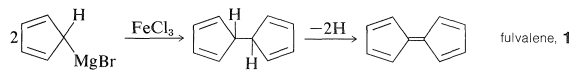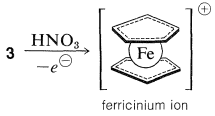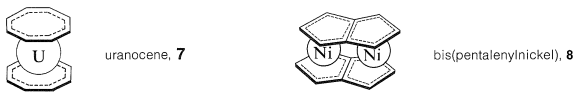# 31.1: Metallocenes

$$\newcommand{\vecs}{\overset { \rightharpoonup} {\mathbf{#1}} }$$ $$\newcommand{\vecd}{\overset{-\!-\!\rightharpoonup}{\vphantom{a}\smash {#1}}}$$$$\newcommand{\id}{\mathrm{id}}$$ $$\newcommand{\Span}{\mathrm{span}}$$ $$\newcommand{\kernel}{\mathrm{null}\,}$$ $$\newcommand{\range}{\mathrm{range}\,}$$ $$\newcommand{\RealPart}{\mathrm{Re}}$$ $$\newcommand{\ImaginaryPart}{\mathrm{Im}}$$ $$\newcommand{\Argument}{\mathrm{Arg}}$$ $$\newcommand{\norm}{\| #1 \|}$$ $$\newcommand{\inner}{\langle #1, #2 \rangle}$$ $$\newcommand{\Span}{\mathrm{span}}$$ $$\newcommand{\id}{\mathrm{id}}$$ $$\newcommand{\Span}{\mathrm{span}}$$ $$\newcommand{\kernel}{\mathrm{null}\,}$$ $$\newcommand{\range}{\mathrm{range}\,}$$ $$\newcommand{\RealPart}{\mathrm{Re}}$$ $$\newcommand{\ImaginaryPart}{\mathrm{Im}}$$ $$\newcommand{\Argument}{\mathrm{Arg}}$$ $$\newcommand{\norm}{\| #1 \|}$$ $$\newcommand{\inner}{\langle #1, #2 \rangle}$$ $$\newcommand{\Span}{\mathrm{span}}$$$$\newcommand{\AA}{\unicode[.8,0]{x212B}}$$

The discovery of ferrocene was one of those fortuitous accidents that was wholly unforeseeable - the kind of discovery which, over and over again, has changed the course of science. Pauson was trying to synthesize fulvalene, $$1$$, by first coupling two molecules of cyclopentadienylmagnesium bromide with $$\ce{FeCl_3}$$ and then dehydrogenating the product:The rationale for the coupling reaction was that phenylmagnesium bromide with $$\ce{FeCl_3}$$ gives high yields of biphenyl, presumably by way of an unstable phenyliron compound:The reaction product was a beautifully crystalline, highly stable orange substance, $$\ce{C_{10}H_{10}Fe}$$, which Pauson formulated as a simple combination of two cyclopentadienide anions and ferrous ion with two $$\ce{C-Fe}$$ bonds, $$2$$. However, the product soon was shown by a variety of physical methods to have the "sandwich" structure, $$3$$:The bonding between the metal and the cyclopentadiene rings involves the $$\pi$$ electrons of the two rings, all carbons being equally bonded to the central ferrous ion. The latter, in accepting a share of 12 $$\pi$$ electrons from two cyclopentadienyl anions, achieves the 18 outer-shell electron configuration$$^1$$ of the inert gas, krypton. Analysis of the structure of crystalline ferrocene shows that when you look down on the molecule along the ring-iron-ring axis the cyclopentadiene rings are seen to be staggered with respect to one another, as shown in $$4$$. Ferrocene has mp $$173^\text{o}$$ and, although stable to sulfuric acid, it is readily oxidized by nitric acid to the less stable ferricinium ion:Like benzene, ferrocene does not react easily by addition but does undergo electrophilic substitution. For example, Friedel-Crafts acylation (Section 22-4F) with $$\ce{CH_3COCl}$$ gives both a monoethanoylferrocene and a diethanoylferrocene. The two acyl groups become attached to two different rings and, because only one diethanoylferrocene can be isolated, the cyclopentadienyl groups appear to be free to rotate about the axis of the carbon-iron bonds:Ferrocene is only one of a large number of compounds of transition metals with the cyclopentadienyl anion. Other metals that form sandwich-type structures similar to ferrocene include nickel, titanium, cobalt, ruthenium, zirconium, and osmium. The stability of metallocenes varies greatly with the metal and its oxidation state; ferrocene, ruthenocene, and osmocene are particularly stable because in each the metal achieves the electronic configuration of an inert gas. Almost the ultimate in resistance to oxidative attack is reached in $$\ce{(C_2H_5)_2Co}^\oplus$$, cobalticinium ion, which can be recovered from boiling aqua regia (a mixture of concentrated nitric and hydrochloric acids named for its ability to dissolve platinum and gold). In cobalticinium ion, the metal has the 18 outer-shell electrons characteristic of krypton.

Many other unsaturated organic compounds can form $$\pi$$ complexes with transition metals. A substance that is in some ways analogous to ferrocene is the complex of two benzene molecules with chromium metal, called dibenzenechromium. The bonding involves zerovalent chromium and the $$\pi$$ electrons of the two benzene rings. In dibenzenechromium, the electronic configuration of the chromium atom is similar to that of krypton:Although dibenzenechromium is thermally quite stable, it is less so than ferrocene and melts with decomposition at $$285^\text{o}$$ to give benzene and metallic chromium. Furthermore, it appears to lack the aromatic character of either benzene or ferrocene as judged by the fact that it is destroyed by reagents used for electrophilic substitution reactions.

Several transition-metal complexes of cyclobutadiene have been prepared, and this is all the more remarkable because of the instability of the parent hydrocarbon. Reactions that logically should lead to cyclobutadiene give dimeric products instead. Thus, 3,4-dichlorocyclobutene has been dechlorinated with lithium amalgam in ether, and the hydrocarbon product is a dimer of cyclobutadiene, $$5$$. However, 3,4-dichlorocyclobutene reacts with diiron nonacarbonyl, $$\ce{Fe_2(CO)_9}$$, to give a stable iron tricarbonyl complex of cyclobutadiene, $$6$$, whose structure has been established by x-ray analysis. The $$\pi$$-electron system of cyclobutadiene is considerably stabilized by complex formation with iron, which again attains the electronic configuration of krypton.Oxidation of $$6$$ with ceric iron, $$\ce{Ce}$$(IV), releases cyclobutadiene which quickly dimerizes, but can be trapped by good dienophiles such as ethyl propynoate to give a cycloadduct.

Many metallocene derivatives are known of other conjugated cyclic polyenes. Examples are bis(cyclooctatetraene)uranium (uranocene, $$7$$) and bis(pentalenylnickel), $$8$$ (see Section 22-12B):Many of the metallocene compounds display unusual reactivities and reactions, of which none is more startling than the discovery by the Russian chemist, M. E. Vol'pin, of the absorption of dinitrogen, $$\ce{N_2}$$, by titanocene, $$\ce{(C_2H_5)_2Ti}$$, to form a complex or complexes that can be reduced easily to form ammonia. The nature of these complexes is in doubt, but very clear evidence has been obtained by J. E. Bercaw for the structure of the complex $$9$$ formed from decamethylzirconocene and dinitrogen:This complex treated with acids gives $$\ce{NH_2-NH_2}$$ and some $$\ce{NH_3}$$.

$$^1$$Figure 6-4 shows that iron(0) has 8 electrons in the $$4s$$ and $$3d$$ orbitals. Ferrous iron $$\left( \ce{Fe^{2+}} \right)$$ then will have 6 outer-shell electrons. This 6 plus the 12 $$\pi$$ electrons of the two cyclopentadienide rings makes the 18-electron total and the krypton electronic configuration.